1. Worksheets>
2. Math>
3. Pre-Algebra>
4. Integers>
5. Addition and Subtraction

Integers: Addition and Subtraction Worksheets

Integer worksheets have a vast collection of printable worksheets based on addition and subtraction of integers. This web page includes integer addition chart, addition squares, in-and-out boxes, word problems, subtraction of integers, reading thermometer and more.

Display charts can be used in classrooms to teach addition of integers. Review integer addition by using these blank charts.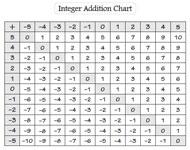Display chart:

Blank chart:

Find the sum of the integers in these worksheets. Use the correct sign while adding a positive integer with a negative integer.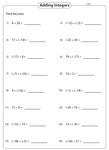Level 1:

Level 2:

Find the sum by adding the integers in the 1st row with the integers in the 1st column and fill them in the squares provided.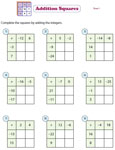2x2:

3x3:

Adding 3 or 4 integers

Use these free integer worksheets to practice addition of 3 or 4 integers. Each worksheet has ten problems.Subtracting integers

In these subtracting integers worksheets, find the difference of the integers'. Six exclusive worksheets are here for practice.Level 1:

Level 2:

Adding and subtracting integers

Add or subtract the integers to find the sum or difference in the following worksheets.Level 1:

Level 2:

Missing integers: Type 1

Find the missing integer in each equation where the sum or difference is always equal to zero.Missing integers: Type 2

Find the missing integer in the following addition and subtraction equations. Integers ranging from -20 to +20 are given in these worksheets.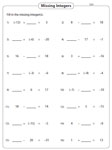Addition and subtraction: Thermometer

The temperature on each thermometer rises or drops by few degrees. Find the new reading in each thermometer.These worksheets consist of word problems based on real time applications like temperature, radar speed signs and account transactions.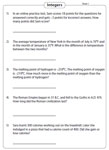Simplify

Simplify the expressions involving addition and subtraction of integers. There are ten problems in each worksheet.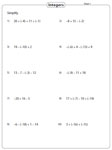In-and-out boxes: Add and subtract

Follow the rule in each table and fill in the in-and-out boxes. Each rule is based either on addition or subtraction of integers.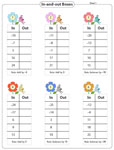Evaluate: Add and subtract

Substitute the values of the unknown variables in the given expressions and evaluate them. Use the addition and subtraction operation.The entire collection of worksheets on adding and subtracting integers can be downloaded in a jiffy!